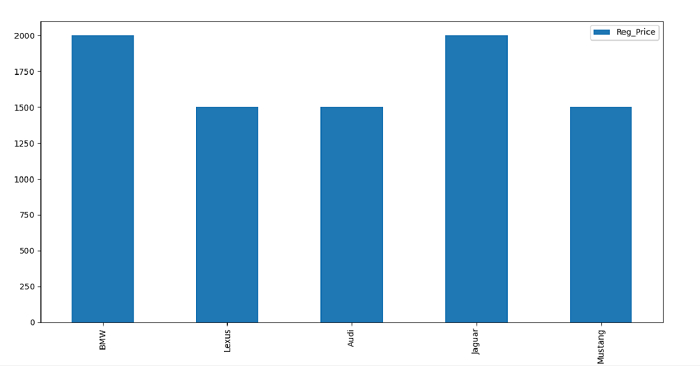# Python - How to plot a Pandas DataFrame in a Bar Graph

Let’s say the following are the contents of our CSV file −

       Car   Reg_Price
0      BMW        2000
1    Lexus        1500
2     Audi        1500
3   Jaguar        2000
4  Mustang        1500

Import the required libraries −

import pandas as pd
import matplotlib.pyplot as mp

Our CSV file is on the Desktop. Load data from a CSV file into a Pandas DataFrame −

d = pd.read_csv("C:\Users\amit_\Desktop\SalesData.csv")

dataFrame = pd.DataFrame(d.head(), columns=["Car","Reg_Price"])

Plot the DataFrame −

dataFrame.plot(x="Car", y="Reg_Price", kind="bar", figsize=(10, 9))


## Example

Following is the code −

import pandas as pd
import matplotlib.pyplot as mp

# dataframe

# plotting the dataframe
dataFrame.plot(x="Car", y="Reg_Price", kind="bar", figsize=(10, 9))

# displaying bar graph
mp.show()

## Output

This will display the following output −